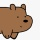Home
IT Knowledge
Inspiration
Languages
EN

# TypeScript - constructor overloads / multiple constructors / many constructors

25 points
Created by:Palus-Bear
646

## 1. Problem

Needed two or more constructors.

``````class MyClassName {

public constructor(a : number);
public constructor(a : number, b : number);
}``````

## 2. Solution

In TypeScript, constructor overloading looks different way than in C++, Java, or C#. The main idea to overload the constructor is to create a common constructor that checks what kind of parameters were passed to create an object and later do some logic for the proper case. Useful is to add constructors' definitions to help other programmers to know how to use class in a proper way.

### 2.1. Optional argument example

In this section `?` operator was used to create a common constructor.

``````class MyClassName {

//public constructor(a: number);
//public constructor(a: number, b: number);

public constructor(a: number, b?: number) {

if (b === undefined) {
console.log('Used constructor 1');
} else {
console.log('Used constructor 2');
}
}
}``````

### 2.2. Common constructor example

In this section, we used an array to describe a common constructor.

``````class MyClassName {

public constructor(a : number);
public constructor(a : number, b : number);

public constructor(...args: any[]) {

if (args.length === 1) {
console.log('Used constructor 1');
return;
}
if (args.length === 2) {
console.log('Used constructor 2');
return;
}
throw new Error('Undefined constructor.');
}
}``````

### 2.3. Argument with multiple type example

In this section, we used an argument of two types.

``````class MyClassName {

//public constructor(a: number, b: number);
//public constructor(a: number, b: string);

public constructor(a: number, b: number | string) {

if (typeof b === 'number') {
console.log('Used constructor 1');
} else {
console.log('Used constructor 2');
}
}
}``````

## 3. More complicated example

Constructor overloading requires writing some logic to detect, which constructor has been used. `public constructor(...args : Array<any>)` contains common logic, where depending on used arguments special case of the object creating should be used. Using array in common constructor we are able to use totally different constructors with different types in the same place.

``````class Point {
private coordinates : Array<number>;

public constructor(x: number, y: number);
public constructor(x: number, y: number, z: number);
public constructor(x: number, y: number, z: number, ...coordinates: Array<number>);
public constructor(coordinates: Array<number>);

public constructor(...args: Array<any>) { // common logic constructor

if (args.length === 0) {
throw new Error('Arguments are not defined.');
}
let arg = args;
if (Array.isArray(arg)) {
if(arg.length < 2) {
throw new Error('Minimum number of dimmensions is 2.');
}
this.coordinates = arg;
} else {
this.coordinates = args;
}
}

public getCoordinate(dimmension: number): number {
return this.coordinates[dimmension];
}

public toString(): string {
let result = '{';
if (this.coordinates.length > 0) {
result += ' ' + this.coordinates;
for (let i = 1; i < this.coordinates.length; ++i) {
result += ', ' + this.coordinates[i];
}
}
return result + ' }';
}
}

let a = new Point(1, 2);
let b = new Point(1, 2, 3);
let c = new Point(1, 2, 3, 4);
let d = new Point([1, 2]);
let e = new Point([1, 2, 3]);
let f = new Point([1, 2, 3, 4]);

console.log(a.toString());
console.log(b.toString());
console.log(c.toString());
console.log(d.toString());
console.log(e.toString());
console.log(f.toString());``````

Output:

``````{ 1, 2 }
{ 1, 2, 3 }
{ 1, 2, 3, 4 }
{ 1, 2 }
{ 1, 2, 3 }
{ 1, 2, 3, 4 }``````

Note: big number of overloading can lead to big amount of mistakes so it is better to avoid this technic.